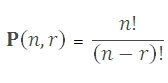# SQL Server PERMUT function

Updated: 9 August 2010

Use PERMUT to calculate the number of permutations for a given number objects that can be selected from a number of objects. Use PERMUT to calculate combinations where the ordering of objects is significant. Use BICO where the ordering of objects is not significant.Syntax
SELECT [wctStatistics].[wct].[PERMUT] (
<@Number, float,>
,<@Number_chosen, float,>)
Arguments
@Number
is any positive number describing the number of objects. @Number is an expression of type float or of a type that can be implicitly converted to float
@Number_chosen
is any positive number describing the number of objects in each permutation. @Number_chosen is an expression of type float or of a type that can be implicitly converted to float
Return Types
float
Remarks
·         If @Number ≤ 0, PERMUT returns an error.
·         If @Number_chosen < 0, PERMUT returns an error.
·         Both @Number and @Number_chosen are truncated to zero decimal places.
Examples

Select
wct.PERMUT(14, 3)

This produces the following result
----------------------
2184

(1 row(s) affected)

### SupportCopyright 2008-2023 Westclintech LLC         Privacy Policy        Terms of Service